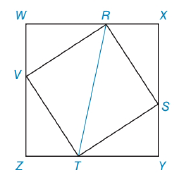Chapter 5.4, Problem 41EElementary Geometry For College St...

7th Edition
Alexander + 2 others
ISBN: 9781337614085

Solutions

Chapter
SectionElementary Geometry For College St...

7th Edition
Alexander + 2 others
ISBN: 9781337614085
Textbook Problem

In the figure, square RSTV has its vertices on the sides of square WXYZ as shown. If ZT=5 and TY=12 find TS. Also find RT.To determine

To Find:

The length of TS and RT.

Explanation

Given In the figure, square RSTV has its vertices on the sides of square WXYZ as shown. If ZT=5 and TY=12.

Since WXYZ is a square, we obtain Y and Z are right angles. So,

mY=mZ=900.

And since RSTV is also a square, VTS is a right angle VTS=900,

By the Angle-Addition Postulate we see,

mZTY=mVTS+mVTZ+mSTY         =900+mVTZ+mSTY         =1800

The sum of angles in a triangle is 1800.

From ΔSYT

mSTY+mTSY+mY=1800mSTY+mTSY+900=1800mSTY+mTSY=900,

Then,

mYST=mZTV

Still sussing out bartleby?

Check out a sample textbook solution.

See a sample solution

The Solution to Your Study Problems

Bartleby provides explanations to thousands of textbook problems written by our experts, many with advanced degrees!

Get Started

Evaluate the integral, if it exists. 12(8x3+3x2)dx

Single Variable Calculus: Early Transcendentals

Perform the indicated operation for the following. 22.

Contemporary Mathematics for Business & Consumers

Maximize P=x+3y Subjected to 2x+3y113x+7y24x0,y0

Finite Mathematics for the Managerial, Life, and Social Sciences

2 1 0 does not exist

Study Guide for Stewart's Multivariable Calculus, 8th

Evaluate ∫16t3 ln t dt. 4t4 + C 4t3 ln t − 16t3 + C 4t4 − t3 ln t + C 4t4 ln t − t4 + C

Study Guide for Stewart's Single Variable Calculus: Early Transcendentals, 8th